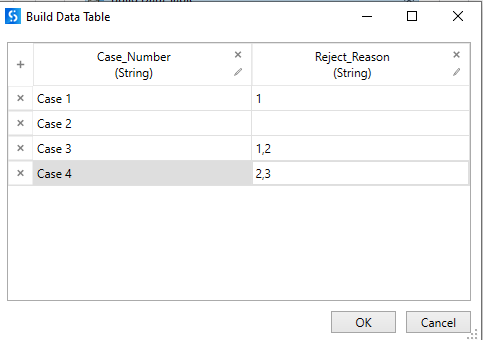# Need a help to write a LINQ query for a datatable

Hi Team,

Need your help with LINQ Query for the below datatable(this is a subset of datatable):First I need to identify case numbers having multiple reasons like Case 3 and Case 4; if yes then read those reasons. On the basis of particular reason i need to read another column from Original datatable

I know it’s bit complex and confusing. I know how we do that with for each, but we have more than 10K records. So want to use LINQ query or any other method which would be faster.

Thanks!!

HI,

Can you share expected output?
Is there another datatable (reason mastrer?) isn’t it? Can you also share it?

Regards,

Linq query is not faster than filter datatable activity. That is a misconception. There are other methods also which can outperform Linq or Filter Datatable activity.

Refer this thread for the benchmark.

Hi @Yoichi/ @jeevith,

So here is the screenshot of datatable and required columns which i need to read on the basis of reject reason:

SO need to find the rows with multiple Reject Reason and on the basis of particular reject reason need to read other columns as well.

Hope this helps to understand the requirement!!

Thanks!

HI,

Can you share expected output for the above input, if possible?

Regards,

Hi,

``````dict = dtMaster.AsEnumerable.ToDictionary(Function(r) r("Column").ToString,Function(r) r("Pattern").ToString)
``````

then

``````dt =dt.AsEnumerable.Where(Function(r) Not String.IsNullOrEmpty(r("Reject_Reasons").ToString)).Select(Function(r) dt.Clone.LoadDataRow({r("Case_Number"), _
If(System.Text.RegularExpressions.Regex.IsMatch(r("Reject_Reasons").ToString.ToLower,dict("Billing_Account_Number")),r("Billing_Account_Number"),""), _
If(System.Text.RegularExpressions.Regex.IsMatch(r("Reject_Reasons").ToString.ToLower,dict("Rate_Plan_Name")),r("Rate_Plan_Name"),""), _
If(System.Text.RegularExpressions.Regex.IsMatch(r("Reject_Reasons").ToString.ToLower,dict("Fighting_Fund_Service_Number")),r("Fighting_Fund_Service_Number"),""), _
r("Reject_Reasons")
},False)).CopyToDataTable
``````

This returns the following.

Sample20230302-5L.zip (10.4 KB)

Regards,

HI @Yoichi,

Thanks a lot for the query!!

To get just the rows with multiple reject reasons, i did this change:

``````dt.AsEnumerable.Where(Function(r) Not String.IsNullOrEmpty(r("Reject_Reasons").ToString)).Select(Function(r) dt.Clone.LoadDataRow({If(r("Reject_Reasons").ToString.Contains(","),r("Case_Number"),""), _
If(System.Text.RegularExpressions.Regex.IsMatch(r("Reject_Reasons").ToString.ToLower,dict("Billing_Account_Number")) And r("Reject_Reasons").ToString.Contains(","),r("Billing_Account_Number"),""), _
If(System.Text.RegularExpressions.Regex.IsMatch(r("Reject_Reasons").ToString.ToLower,dict("Rate_Plan_Name")) And r("Reject_Reasons").ToString.Contains(",") , r("Rate_Plan_Name"),""), _
If(System.Text.RegularExpressions.Regex.IsMatch(r("Reject_Reasons").ToString.ToLower,dict("Fighting_Fund_Service_Number")) And r("Reject_Reasons").ToString.Contains(",") ,r("Fighting_Fund_Service_Number"),""), _
If(r("Reject_Reasons").ToString.Contains(","),r("Reject_Reasons"),"")
},False)).CopyToDataTable

``````

This is giving me the below result:

However, I want to understand the logic of code here. I got most of the query what we’re trying to do, the part which i am not sure is:
dt.Clone.LoadDataRow → what this part is doing or if you can explain the complete logic, that would be really helpful for me and my peers. As we all are new to UiPath journey.

Much Thanks!
Deepika

Hi,

LoadDataRow method returns datarow which has items set as argument.
In this case, the updated-datarows are created by LoadDataRow in LINQ Select method, then output datatable by CopyToDataTable.

Please also see the following official document if necessary.

Regards,

This topic was automatically closed 3 days after the last reply. New replies are no longer allowed.